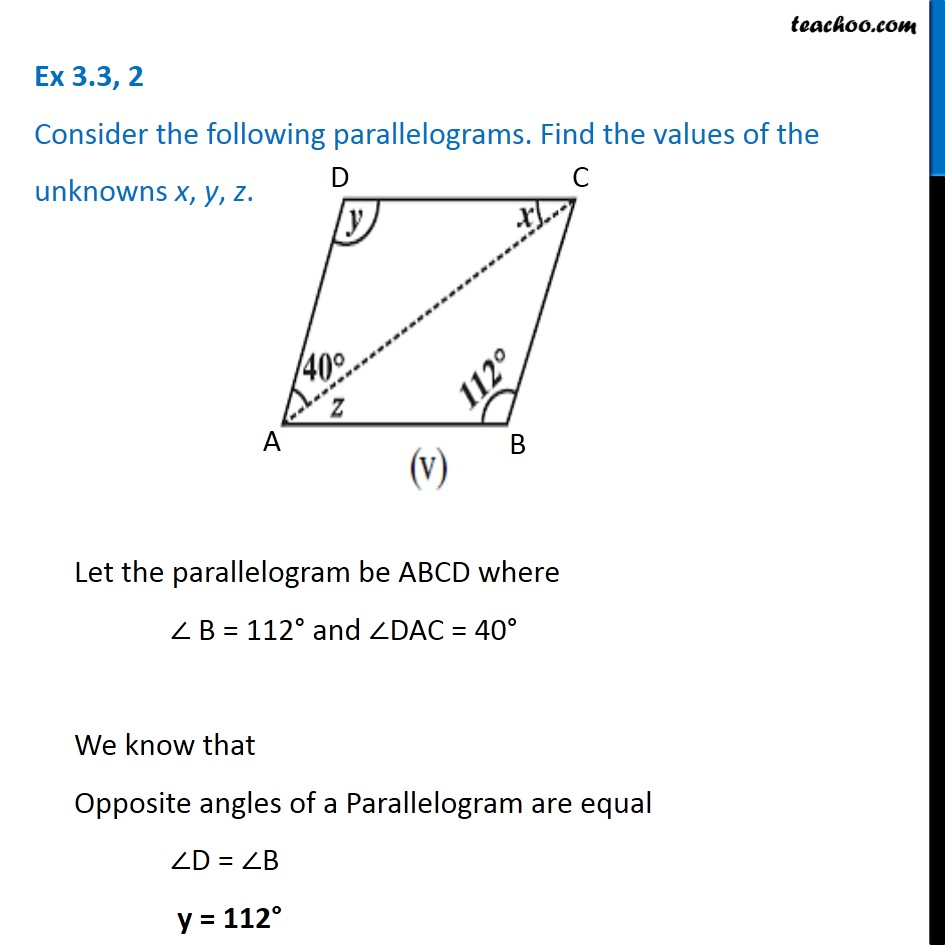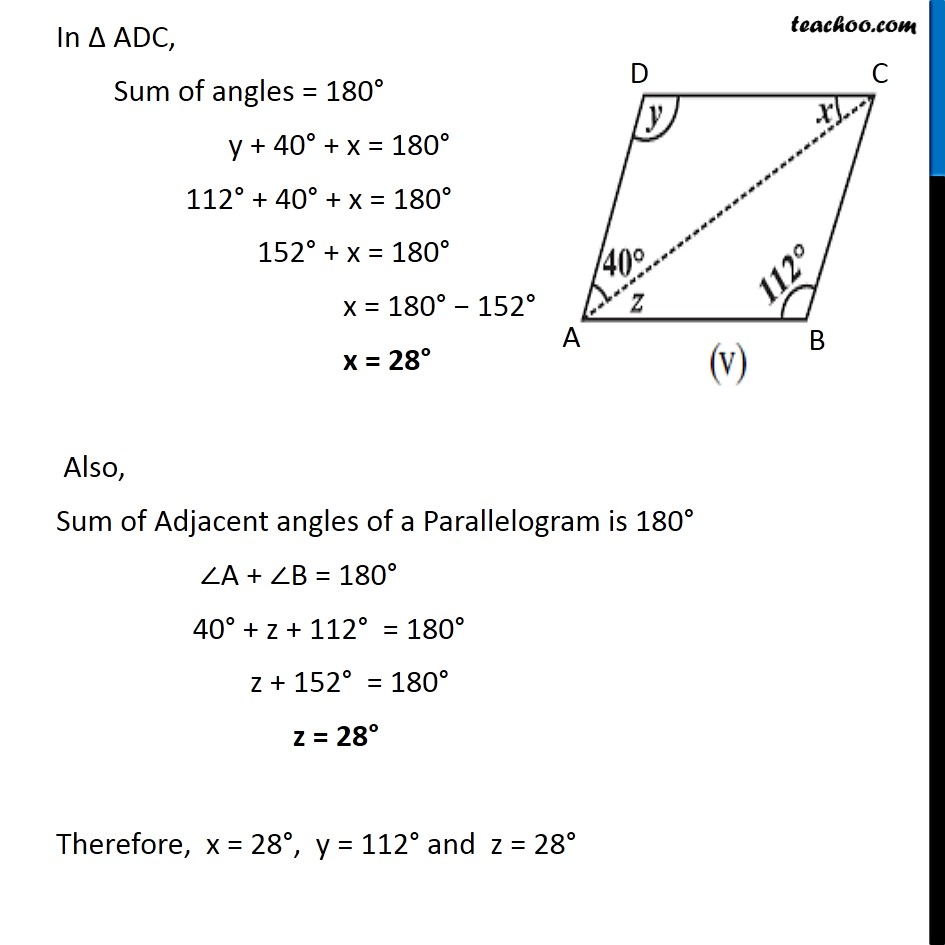Ex 3.3

Chapter 3 Class 8 Understanding Quadrilaterals
Serial order wiseLearn in your speed, with individual attention - Teachoo Maths 1-on-1 Class

### Transcript

Ex 3.3, 2 Consider the following parallelograms. Find the values of the unknowns x, y, z. Let the parallelogram be ABCD where ∠ B = 112° and ∠DAC = 40° We know that Opposite angles of a Parallelogram are equal ∠A = ∠C y = 112° In ∆ ABC, Sum of angles = 180° y + 40° + x = 180° 112° + 40° + x = 180° 152° + x = 180° x = 180° − 152° x = 28° Also, Sum of Adjacent angles of a Parallelogram is 180° ∠A + ∠B = 180° ∠DAC + ∠BAC + ∠B = 180° 40° + z + 112° = 180° z + 152° = 180° z = 28° Therefore, x = 28°, y = 112° and z = 28°